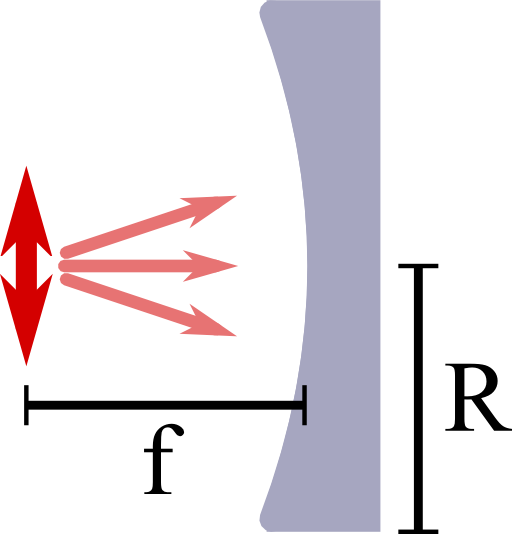## The Directivity of a Parabolic ReflectorWhy are parabolic reflectors as part of antenna systems so common? The reason is that they provide a very high directivity. In this problem you will learn about this very basic concept of antenna theory to quantify emitters and receivers at the examples of a dipole, a "half-dipole" and a parabolic reflector.

## Problem StatementCalculate the directivity of a dipole oscillating in $$z$$  direction. Then, assume the radiation propagating in negative $$x$$ -direction gets absorbed in the farfield of the dipole. How does the directivity change?
Now we want to put this “half-dipole” in the focal point of a parabolic reflector with opening radius $$R$$ and focal distance $$f$$, see figure on the right. How can we calculate the directivity of this device approximately for a small opening angle $$\delta\theta \approx R/f$$ Assume that the backreflected light is not influenced by the “half-dipole” itself and calculate $$D$$ for a wavelength $$\lambda=500\,$$nm and an opening radius $$R=20\,$$cm.

## Background: Directivity in Antenna Theory

The radiated power $$dP_{\mathrm{rad}}$$ into a certain angle $$d\Omega=\sin\theta d\theta d\varphi$$ is related to the radial component of the time-averaged Poynting vector $$S_{r}\left(r,\theta,\varphi\right)$$,$\begin{eqnarray*} dP_{\mathrm{rad}}&=&r^{2}S_{r}\left(r,\theta,\varphi\right)d\Omega\equiv r^{2}S_{r,\mathrm{max}}\left(r\right)F\left(\theta,\varphi\right)d\Omega\ . \end{eqnarray*}$As defined above, one may also normalize $$S_{r}$$ und use the normalized radiation intensity $$F$$ instead. This quantity is used to define the directivity $$D$$ of a certain antenna or source,$\begin{eqnarray*} D&=&\frac{4\pi}{\Omega_{p}\equiv\int_{0}^{2\pi}\int_{0}^{\pi}F\left(\theta,\varphi\right)\sin\theta d\theta d\varphi}\ . \end{eqnarray*}$